## Data Structures and Algorithms 2, Fall 1998 Problem Set 5 (7 - 9 Dec)

1. Determine whether the following propositional formulas are satisfiable:
1.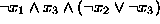;
2.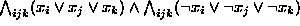.
In formula (b), the indexing is over all triples (i, j, k) such that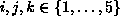and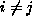,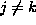,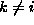.
2. Suppose that SAT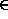P. Show that there then exists a polynomial time algorithm that for each formula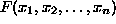either states that the formula is not satisfiable, or actually finds a satisfying truth assignment to the variables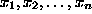. (Hint: It is easy to see that formulais satisfiable, if and only if either formula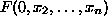or formula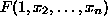is satisfiable.)
3. Prove the following Lemmas (p. 115 of the lecture notes):
1. If L is an NP-complete set and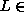P, then P = NP.
2. If L is an NP-complete set,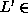NP and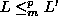, then also L' is NP-complete.
4. Construct a polynomial time reduction from the Clique problem to the Vertex Cover (VC) problem. (Hint: Look at the reductions presented on pp. 111-112 of the lecture notes.)
5. Prove that the following Set Packing problem is NP-complete:
Given a collection of subsets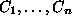of a finite set U, and a natural number k, is it possible to choose a subcollection of at least k disjoint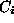's (i.e.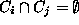for)?
(Hint: Reduction from the Independent Set (IS) problem.)

Reminder: This is the last problem set. The last lecture of the course is on Monday, 7 Dec, and the course exam takes place on Tuesday, 15 Dec (8-12).

Pekka Orponen
Thu Dec 3 15:17:23 EET 1998